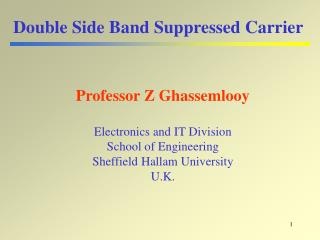DownloadDownload PresentationDouble Side Band Suppressed Carrier

# Double Side Band Suppressed Carrier

Download Presentation## Double Side Band Suppressed Carrier

- - - - - - - - - - - - - - - - - - - - - - - - - - - E N D - - - - - - - - - - - - - - - - - - - - - - - - - - -
##### Presentation Transcript

1. Double Side Band Suppressed Carrier Professor Z Ghassemlooy Electronics and IT Division School of Engineering Sheffield Hallam University U.K.

2. Contents • Theory • Implementation • Transmitter • Detector • Synchronous • Square • Power analysis • Summary

3. Carrier USB LSB c - m c c + m Single frequency Double Side Band Suppressed Carrier From AM spectrum: • Carries signal c carries no information m. • Carries signal consumes a lot of power more than 50% Question: Why transmit carrier at all? Ans: Question: Can one suppress the carrier? Ans.:Yes, just transmit two side bands (i.e DSB-SC) But what is the penalty? System complexity at the receiver

4. upper side band lower side band DSB-SC - Theory General expression: Let k1 = 1, C = 0 and c = 0, the modulated carrier signal, therefore: Information signal m(t) = Em cos mt Thus

5. B = 2m Notice: No carrier frequency DSB-SC - Waveforms Mixer (Multiplier)

6. Ec (1+ 0.5 m(t) cos ct 0.5 m(t) + Carrier Ec cos ct DSB-SC Ecm(t) cos ct - -0.5 m(t) Ec (1- 0.5 m(t) cos ct DSB-SC - Implementation • Balanced modulator AM mod. + AM mod. • Ring modulator • Square-law modulator

7. Low pass filter DSB-SC Local oscillator c(t) = cos ct information Low pass filter m 2c-m 2c+m DSB-SC - Detection • Synchronous detection Multiplier Message signal • Condition: • Local oscillator has the same • frequency and phase as that of the • carrier signal at the transmitter. high frequency

8. Low pass filter DSB-SC Multiplier Message signal Local oscillator c(t) = cos(ct+) information Low pass filter m 2c-m 2c+m DSB-SC - Synchronous Detection • Case 1 - Phase error • Condition: • Local oscillator has the same • frequency but differentphase • compared to carrier signal at the • transmitter. high frequency

9. In-phase yip(t) 0.5Ec m(t) cos  X DSB-SC LPF Ec cos (ct+) Vphase(t) Phase discriminator VCO 90o phase shift Ec sin (ct+) 0.5Ec m(t) sin  X. LPF yqp(t) Quadrature-phase Phase Synchronisation -Costa Loop Recovered signal • When there is no phase error. The quadrature component is zero • When 0, yip(t) decreases, while yqp(t) increases • The out put of the phase discriminator is proportional to 

10. Low pass filter DSB-SC Multiplier Message signal Local oscillator c(t)=Eccos(ct+) information Low pass filter m 2c-m 2c+m DSB-SC - Synchronous Detection • Case 1 - Frequency error • Condition: • Local oscillator has the same • phase but different frequency • compared to carrier signal at the • transmitter. high frequency

11. g(t) Squaring circuit g =x2 Band pass filter C 2c DSB-SC - Square Detection y(t) DSB-SC Si(t)  by 2 Regenerated carrier z(t) Multiplier Message signal Low pass filter g(t) = Si2(t) = B2 cos2mt cos2ct = B2 (½ + ½ cos 2 mt )(½ + ½ cos 2 ct ) = B2/4 [1 + ½ cos 2(c + m)t + ½ cos 2(c - m)t + cos 2mt + cos 2 ct ] y(t) = B2/4 cos 2wct z(t) = B2/4 cos wct

12. DSB-SC - Power • The total power (or average power): • The maximum and peak envelop power

13. DSB-SC - Summary • Advantages: • Lower power consumption • Disadvantage: • - Complex detection • Applications: • - Analogue TV systems: to transmit colour information • - For transmitting stereo information in FM sound broadcast • at VHF